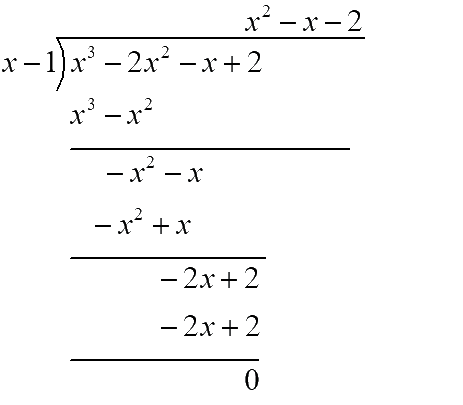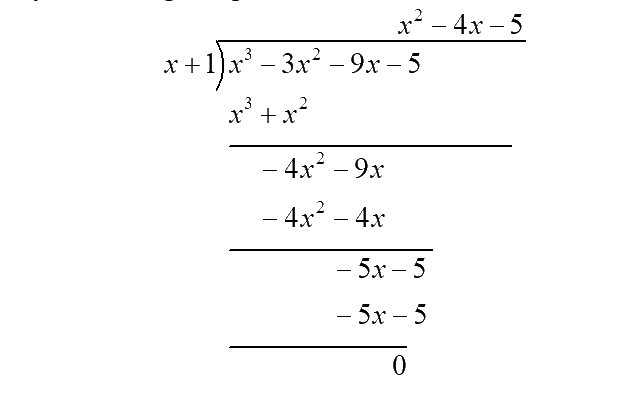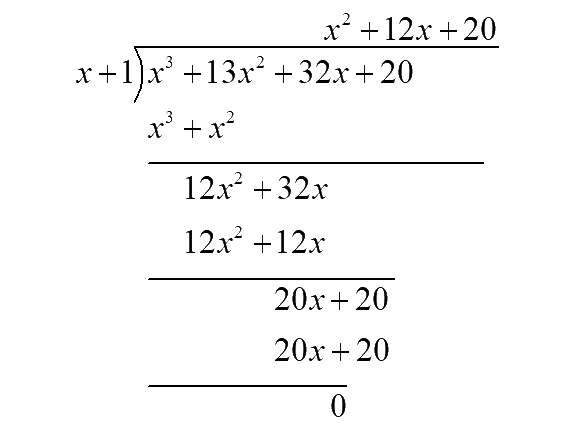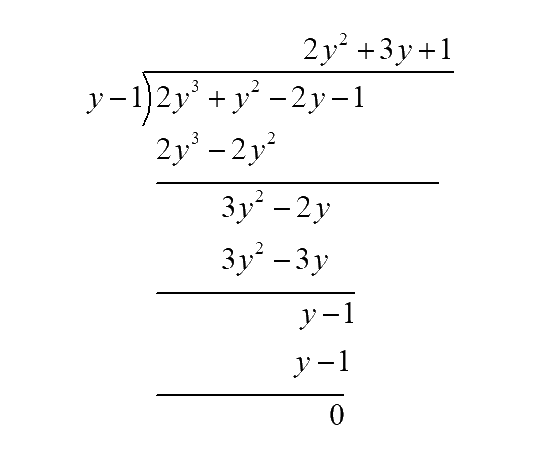# Ex.2.4 Q5 Polynomials Solution - NCERT Maths Class 9

## Question

Factorise:

(i) \begin{align}{x^3} - 2{x^2} - x + 2 \end{align}

(ii) \begin{align}{x^3} - 3{x^2} - 9x - 5\end{align}

(iii) \begin{align}{x^3} + 13{x^2} + 32x + 20 \end{align}

(iv) \begin{align}2{y^3} + {y^2} - 2y - 1\end{align}

## Text Solution

Steps:

(i) Let $$p(x) = {x^3} - 2{x^2} - x + 2$$

By the factor theorem we know that $$x-a$$ is a factor of $$p(x)$$if $$p(a) = 0.$$

We shall find a factor of $$p(x)$$ by using some trial value of $$x,$$ say $$x = 1.$$

\begin{align} p(1) &= {(1)^3} - 2{(1)^2} - 1 + 2\\ &= 1 - 2 - 1 + 2 = 0\end{align}

Since the remainder of $$p(1) = 0$$ , by factor theorem we can say $$x=1$$ is a factor of $$p(x) = {x^3} - 2{x^2} - x + 2.$$

Now divide $$p(x)$$ by $$x-1$$ using long division,Hence $${x^3}\!-\!\! 2{x^2}\! -\! x\!+\!\!2\!=\!(x\!-\!\!1)({x^2}\!-\!x\!-\!\!2)$$

Now taking $${x^2} - x - 2$$ , find $$2$$ numbers $$p, q$$ such that:

(i) $$p + q =$$co-efficient of $$x$$

(ii) $$pq =$$ co-efficient of $${x^2}$$ and the constant term.

$$p + q = - 1$$ (co-efficient of $$x$$)

$$pq = 1 \times - 2 = - 2$$(co-efficient of $${x^2}$$ and the constant term.)

By trial and error method, we get $$p = -2, q = 1.$$

Now splitting the middle term of the given polynomial,

\begin{align} {x^2} - x - 2 &= {x^2} - 2x + x - 2\\ \,\,\,\,\,\,\,\,\,\,\,\,\,\,\,\,\,\,\,\,\,\,\,\, &= x(x - 2) + 1(x - 2)\\ \,\,\,\,\,\,\,\,\,\,\,\,\,\,\,\,\,\,\,\,\,\,\,\, &= (x + 1)(x - 2)\end{align}

$$\therefore\!{x^3}\!\!- \!2{x^2}\!\!-\!x\!+\!2\!=\!(x\!\!-\!1)\!(x\!-\!2)(x\!+\!\!1)$$

Method 2:

\begin{align}{x^3}\!\!-\!2{x^2}\!\!-\!x\!+\!2\!&=\!({x^3}\!\!-\!2{x^2})\!-\!(x\!\!-\!\!2)\\ &= {x^2}(x - 2)\!-\!1(x\! -\!\! 2)\\ &= (x - 2)({x^2} - 1)\\ &= (x\!-\!2)(x\!+\!1)(x\!-\!\! 1) \\&\left( \begin{array}{l}{\text{By using }}{a^2} - {b^2}\\ = (a + b)(a - b)\end{array} \right)\end{align}

(ii) Let $$p(x) = {x^3} - 3{x^2} - 9x - 5$$

By the factor theorem we know that $$x-a$$ is a factor of $$p(x)$$ if $$p(a) = 0.$$

We shall find a factor of $$p(x)$$ by using some trial value of $$x,$$ say $$x = 1.$$

\begin{align}p(1) &= {(1)^3} - 3{(1)^2} - 9(1) - 5 \\ &= 1 - 3 - 9 - 5\\ &= - 16 \ne 0\end{align}

Since the remainder of $$p(1) \ne 0$$ , by factor theorem we can say $$x=1$$ is not a factor of $$p(x) = {x^3} - 3{x^2} - 9x - 5.$$

Now say $$x = -1.$$

\begin{align} p(\!-\!1) &\!=\!\!{(\!-\!1)^3}\!-\!\!3{( -\!1)^2}\!\!-\!\! 9(\!-\!1)\!-\!\!5 \\ &= - 1 - 3 + 9 - 5\\ &= - 9 + 9 = 0\end{align}

Since the remainder of $$p( - 1) = 0$$ , by factor theorem we can say $$x=-1$$ is a factor of $$p(x) = {x^3} - 3{x^2} - 9x - 5.$$

Now dividing $$p(x)$$ by $$x+1$$using long division.Hence $${x^3} -\!\!3{x^2}\!\!-\!\!9x\!-\!\!5\!\!=\!\!(x\!+\!1)\!({x^2}\!-\!4x\!-\!5)$$

Now taking $${x^2} - 4x - 5$$ , find $$2$$ numbers $$p, q$$ such that:

(i) $$p + q =$$ co-efficient of $$x$$

(ii) $$pq =$$ co-efficient of $${x^2}$$ and the constant term.

$$p + q = - 4$$ (co-efficient of $$x$$)

$$pq = 1 \times - 5 = - 5$$ (co-efficient of $${x^2}$$ and the constant term.)

By trial and error method, we get $$p = -5, q = 1.$$

Now splitting the middle term of the given polynomial,

\begin{align} {x^2} - 4x - 5 &= {x^2} - 5x + x - 5\\ \,\,\,\,\,\,\,\,\,\,\,\,\,\,\,\,\,\,\,\,\,\,\,\, &= x(x - 5) + 1(x - 5)\\ \,\,\,\,\,\,\,\,\,\,\,\,\,\,\,\,\,\,\,\,\,\,\,\, &= (x + 1)(x - 5) \end{align}

\begin{align}&\therefore {x^3}\!-\!\!2{x^2}\!-\!\!x\!+\!2 \\&= (x\!+\!1)(x\!-\!5)(x\!+\!1)\\ &= \,{(x\!+\!1)^2}\!(x\!- \!\!5)\,\, \end{align}

(iii) Let $$p(x) = {x^3} + 13{x^2} + 32x + 20$$

By the factor theorem we know that $$x-a$$ is a factor of $$p(x)$$ if $$p(a) = 0.$$

We shall find a factor of $$p(x)$$ by using some trial value of $$x,$$ say $$x = -1.$$ (Since all the terms are positive.)

\begin{align}\!p(\!-\!1) &\!=\!{(\!- 1)^3}\!+\!\!13{( - 1)^2}\!\!+\!32( - 1)\!+\!\!20 \\ &= - 1 + 13 - 32 + 20\\ &= 0 \end{align}

Since the remainder of $$p( - 1) = 0$$ , by factor theorem we can say $$x= -1$$ is a factor of $$p(x) = {x^3} + 13{x^2} + 32x + 20.$$

Now dividing $$p(x)$$ by $$x+1$$ using long division,$\left[ \begin{array}{l}\therefore {x^3} + 13{x^2} + 32x + 20\\ = \,(x + 1)({x^2} + 12x + 20)\end{array} \right]$

Now taking $${x^2} + 12x + 20$$ , find $$2$$ numbers $$p, q$$ such that:

(i) $$p + q =$$ co-efficient of $$x$$

(ii) $$pq =$$ co-efficient of $${x^2}$$ and the constant term.

$$p + q = 12$$ (co-efficient of $$x$$)

$$pq = 1 \times 20 = 20$$ (co-efficient of $${x^2}$$ and the constant term.)

By trial and error method, we get $$p = 10, q = 2.$$

Now splitting the middle term of the given polynomial,

\begin{align} {x^2}\!\!+\!12x\!+\!20\!&=\!{x^2}\!\!+\!10x\!+\!\!2x\!+\!\!20\\ &=\!x(x\!+\!\!10)\!+\!\!2(x\!+\!\!10)\\ &= (x\!+\!\!10)\!(x\!+\!\!2)\end{align}

$\left( \begin{array}{l}\therefore {x^3} + \,13{x^2} + \,32x\, + \,20\\ = (x + 1)\,(x + 10)\,(x + 2)\end{array} \right)$

Method 2:

\begin{align}&{x^3} + 13{x^2} + 32x + 20 \\&=\!{x^3}\!+\!10{x^2}\!+\!3{x^2}\!+\!30x\!+\!2x\!+\!20\\ &= {x^2}\!(x\!+\!\!10)\!+\!\!3x(x\!+\!10)\!+\!\!2(\!x\!+\!10\!) \\ &= (x + 10)({x^2} + 3x + 2)\\ &= (x + 10)({x^2} + 2x + x + 2) \\ &= (x + 10)[x(x + 2) + 1(x + 2)]\\ &= (x + 10)(x + 2)(x + 1)\end{align}

(iv) Let  $$p(y) = 2{y^3} + {y^2} - 2y - 1$$

By the factor theorem we know that $$(y-a)$$ is a factor of $$p(y)$$ if $$p(a) = 0.$$

We shall find a factor of $$p(y)$$ by using some trial value of $$y,$$ say $$y = 1.$$

\begin{align} p(1) &= 2{(1)^3} + {(1)^2} - 2(1) - 1\\ \,\,\,\,\,\,\,\,\,\,\,\, &= 2 + 1 - 2 - 1\\ \,\,\,\,\,\,\,\,\,\,\,\, &= 0 \end{align}

Since the remainder of $$p(1) = 0$$ , by factor theorem we can say $$y-1$$ is a factor of $$p(y) = 2{y^3} + {y^2} - 2y - 1$$

Now dividing $$p(y)$$ by $$y-1$$ using long division,$$\therefore\!2{y^3}\!+\!{y^2}\!-\!2y\!-\!\!1\!\!=\!\!(y\!\!-\!\!1)\!(2{y^2}\!+\!3y\!+\!1)$$

Now taking $$2{y^2} + 3y + 1$$ , find $$2$$ numbers $$p, q$$ such that:

(i) $$p + q =$$co-efficient of $$y$$

(ii) $$pq =$$ co-efficient of $${y^2}$$ and the constant term.

$$p + q = 3$$ (co-efficient of $$y$$)

$$pq = 2 \times 1 = 2$$(co-efficient of $${y^2}$$ and the constant term.)

By trial and error method, we get $$p = 2, q = 1.$$

Now splitting the middle term of the given polynomial,

\begin{align} 2{y^2} + 3y + 1 &= 2{y^2} + 2y + y + 1 \\ &= 2y(y + 1) + 1(y + 1)\\&= (2y + 1)(y + 1)\end{align}

$$\!\therefore\!2{y^3}\!+\!\!{y^2}\!-\!2y\!-\!\!1\!\!=\!\!(y\!-\!1)(2y\!+\!\!1)\!(y\!+\!\!1)$$

Video Solution
Polynomials
Ex 2.4 | Question 5
Learn from the best math teachers and top your exams

• Live one on one classroom and doubt clearing
• Practice worksheets in and after class for conceptual clarity
• Personalized curriculum to keep up with school